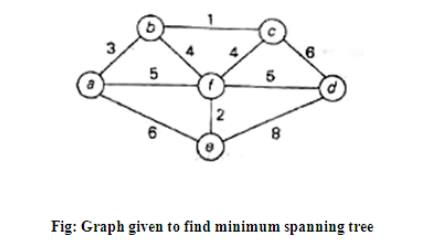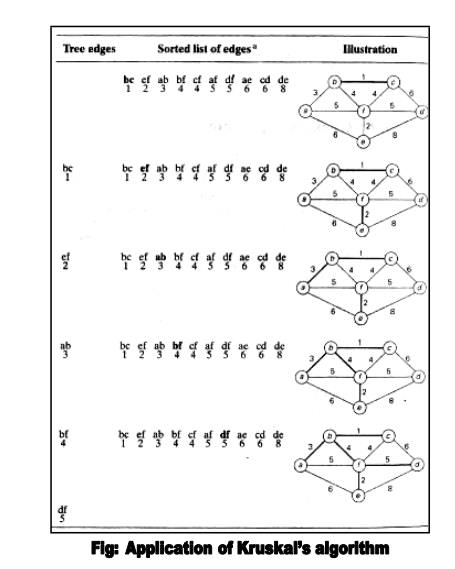Home | | User Interface Design | Spanning Tree Algorithm: PrimÔÇİs and KruskalÔÇİs Algorithm

# Spanning Tree Algorithm: PrimÔÇİs and KruskalÔÇİs Algorithm

Suppose G = (V, E) is a connected graph in which each edge (u, v) in E has a cost c(u, v) attached to it. The cost of a spanning tree is the sum of the costs of the edges in the tree.

SPANNING TREES

A spanning tree  for G is a free tree that connects all the vertices in         V

1. MINIMUM-COST SPANNING TREES

Suppose G = (V, E) is a connected graph in which each edge (u, v) in E has a cost c(u, v) attached to it. The cost of a spanning tree is the sum of the costs of the edges in the tree.

A typical application for minimum-cost spanning trees occurs in the design of communications networks. The vertices of a graph represent cities and the edges possible communications links between the cities. The cost associated with an edge represents the cost of selecting that link for the network. A minimum-cost spanning tree represents a communications network that connects all the cities at minimal cost.

2. The MST Property

There are several different ways to construct a minimum-cost spanning tree. Many of these methods use the following property of minimum-cost spanning trees, which we call the MST property

Let G = (V, E) be a connected graph with a cost function defined on the edges. Let U be some proper subset of the set of vertices V. If (u, v) is an edge of lowest cost such that u

U and v Ôêê V-U, then there is a minimum-cost spanning tree that includes (u, v) as an edge. The proof hat every minimum-cost spanning tree satisfies the MST property is not hard.

Suppose to the contrary that there is no minimum-cost spanning tree for G that includes (u, v). Let T be any minimum-cost spanning tree for G. Adding (u, v) to T must introduce a cycle, since T is a free tree and therefore satisfies property (2) for free trees. This cycle involves edge (u, v). Thus, there must e another edge (u', v') in T such that u' Ôêê U and v' Ôêê

V-U, as illustrated in Fig. 7.5. If not, there could be no way for the cycle to get from u to v

without following the edge (u, v) a second time. Deleting the edge (u', v') breaks the

cycle and yields a spanning tree T' whose

3. PRIMÔÇÖS ALGORITHM

Definition:

spanning tree of a connected graph is its connected acyclic subgraph (i.e., a tree) that contains all the vertices of the graph. A minimum spanning tree of a weighted connected graph is its spanning tree of the smallest weight, where the weight of a tree is defined as the sum of the weights on all its edges. The minimum spanning tree problem is the problem of finding a minimum spanning tree for a given weighted connected graph.

Two serious difficulties to construct Minimum Spanning Tree

1.     The number of spanning trees grows exponentially with the graph size (at least for dense graphs).

2.   Generating all spanning trees for a given graph is not easy.Figure: Graph and its spanning trees; T1 is the Minimum Spanning Tree

PrimÔÇİs algorithm constructs a minimum spanning tree through a sequence of expanding subtrees. The initial subtree in such a sequence consists of a single vertex selected arbitrarily from the set V of the graphÔÇİs vertices.

On each iteration, we expand the current tree in the greedy manner by simply attaching to it the nearest vertex not in that tree. The algorithm stops after all the graphÔÇİs vertices have been included in the tree being constructed.

Since the algorithm expands a tree by exactly one vertex on each of its iterations, the total number of such iterations is n-1, where n is the number of vertices in the graph. The tree generated by the algorithm is obtained as the set of edges used for the tree expansions.

The nature of PrimÔÇİs algorithm makes it necessary to provide each vertex not in the current tree with the information about the shortest edge connecting the vertex to a tree vertex.

We can provide such information by attaching two labels to a vertex: the name of the nearest tree vertex and the length (the weight) of the corresponding edge.

Vertices that are not adjacent to any of the tree vertices can be given the label indicating their ÔÇòinfiniteÔÇû distance to the tree vertices a null label for the name of the nearest tree vertex.

With such labels, finding the next vertex to be added to the current tree T = (VT, ET) become simple task of finding a vertex with the smallest distance label in the set V - VT. Ties can be broken arbitrarily.

After we have identified a vertex u* to be added to the tree, we need to perform two operations:

o Move u* from the set VÔÇöVT to the set of tree vertices VT.

o For each remaining vertex U in VÔÇöVT - that is connected to u* by a shorter edge than the uÔÇİs current distance label, update its labels by u* and the weight of the edge between u* and u, respectively.

Pseudocode of this algorithm4. KRUSKALÔÇÖS ALGORITHM

This is another greedy algorithm for the minimum spanning tree problem that also always yields an optimal solution.

KruskalÔÇİs algorithm looks at a minimum spanning tree for a weighted connected graph G = {V, E} as an acyclic subgraph with |V|-1 edges for which the sum of the edge weights is the smallest. Consequently, the algorithm constructs a minimum spanning tree as an expanding sequence of subgraphs, which are always acyclic but are not necessarily connected on the intermediate stages of the algorithm.The correctness of KruskalÔÇİs algorithm can be proved by repeating the essential steps of the proof of PrimÔÇİs algorithm. The fact that ET is actually a tree in PrimÔÇİs algorithm, but generally just an acyclic subgraph in KruskalÔÇİs algorithm turns out to be an obstacle that can be overcome.

Applying PrimÔÇİs and KruskalÔÇİs algorithms to the same small graph by hand may create an impression that the latter is simpler than the former. This impression is wrong because, on each of its iterations, KruskalÔÇİs algorithm has to check whether the addition of the next edge to the edges already selected would create a cycle.

It is not difficult to see that a new cycle is created if and only if the new edge connects two vertices already connected by a path, i.e., if and only if the two vertices belong to the same connected component. Note also that each connected component of a subgraph generated by KruskalÔÇİs algorithm is a tree because it has no cycles.Study Material, Lecturing Notes, Assignment, Reference, Wiki description explanation, brief detail
Analysis and Design of Algorithm : Traversals, Branch and Bound : Spanning Tree Algorithm: PrimÔÇİs and KruskalÔÇİs Algorithm |